0
169

# IIFT Coding-Decoding Questions PDF [Important]

Practice IIFT solved Coding-Decoding Questions paper tests, which are the practice question to have a firm grasp on the Coding-Decoding topic in the IIFT exam. In this article, one can find the top 20 very Important Coding-Decoding Questions for IIFT based on asked questions in previous exam papers. The IIFT question papers contain actual questions asked with detailed solutions. Download these important Coding-Decoding Questions for IIFT PDF with detailed solutions. Click on the link given below to download the PDF.

Question 1: ‘TQUS’ is related to ‘UPVR’ in the same way as ‘FIRE’ is related to

a) GHSD

b) GIRG

c) GIRF

d) GHRD

Solution:

Given, TQUS is related to UPVR

The logic here is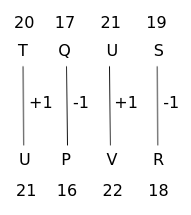Similarly,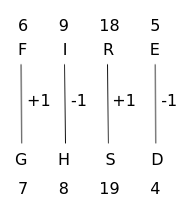$\therefore\$FIRE is related to GHSD in the same way TQUS is related to UPVR

Hence, the correct answer is Option A

Question 2: In certain code language, ‘TELEVISION’ is written as ‘OPJTJWFMFU’. How will ‘REMOTE’ be written as in that language?

a) SFNPUF

b) PUPFNS

c) FUPNFS

d) ETOMER

Solution:

Given, TELEVISION is written as OPJTJWFMFU in the code language

The logic here is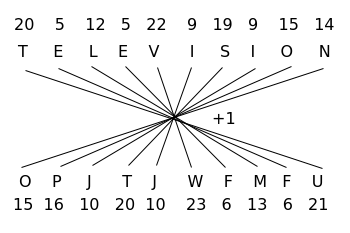Similarly,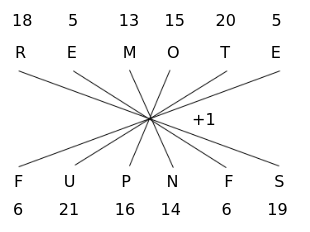$\therefore\$REMOTE is written as FUPNFS in the code language.

Hence, the correct answer is Option C

Question 3: In a certain code language, ‘TRAFFIC’ is written as ‘VTCHHKE’. How will ‘POLICE’ be written as in that language?

a) RQNKEG

b) RMNGEC

c) ONKHBD

d) RMIGEC

Solution:

Given, TRAFFIC is written as VTCHHKE in the code language

The logic here is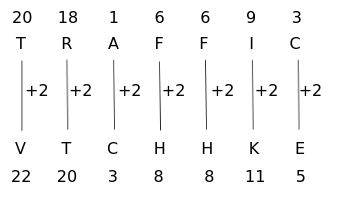Similarly,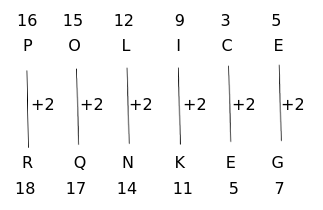$\therefore\$POLICE is written as RQNKEG in the code language.

Hence, the correct answer is Option A

Question 4: In a certain code, ‘sky is blue’ is written as ‘815’ ; ‘blue and red’ is written as ‘725’; ‘Ram likes red’ is coded as ‘203’; ‘Sita and Ram’ is written as ‘379’. What will be the code for ‘Ram likes blue’?

a) 257

b) 512

c) 573

d) 503

Solution:

In the code language,

‘sky is blue’ is written as ‘815’ ……….(1)

‘blue and red’ is written as ‘725’ …….(2)

‘Ram likes red’ is coded as ‘203’ …….(3)

‘Sita and Ram’ is written as ‘379’ …….(4)

Comparing (1) and (2), the code for ‘blue’ is ‘5’

Comparing (3) and (4), the code for ‘Ram’ is ‘3’

Comparing (2) and (3), the code for ‘red’ is ‘2’

From (3), the code for remaining word ‘likes’ is ‘0’

$\therefore\$The code for ‘Ram likes blue’ is ‘503’

Hence, the correct answer is Option D

Question 5: In a certain code language, ‘WORKSHOP’ is written as ‘231518118191211’. How will ‘NOVEMBER’ be written as in that language?

a) 13172251325229

b) 14142251424228

c) 14152251425229

d) 11151251422227

Solution:

Given, WORKSHOP is written as 231518118191211 in the code language.

WORKSHOP $\longrightarrow\$ 23 15 18 11 8 19 12 11

The logic here is

W = 23 $\longrightarrow\$ 23

O = 15 $\longrightarrow\$ 15

R = 18 $\longrightarrow\$ 18

K = 11 $\longrightarrow\$ 11

S opposite is H $\longrightarrow\$ H = 8 $\longrightarrow\$ 8

H opposite is S $\longrightarrow\$ S = 19 $\longrightarrow\$ 19

O opposite is L $\longrightarrow\$ L = 12 $\longrightarrow\$ 12

P opposite is K $\longrightarrow\$ K = 11 $\longrightarrow\$ 11

For the first four letters of the word, value of the letters is coded. For the last four letters of the word, value of the opposite letters is coded.

Similarly,

N = 14 $\longrightarrow\$ 14

O = 15 $\longrightarrow\$ 15

V = 22 $\longrightarrow\$ 22

E = 5 $\longrightarrow\$ 5

M opposite is N $\longrightarrow\$ N = 14 $\longrightarrow\$ 14

B opposite is Y $\longrightarrow\$ Y = 25 $\longrightarrow\$ 25

E opposite is V $\longrightarrow\$ V = 22 $\longrightarrow\$ 22

R opposite is I $\longrightarrow\$ I = 9 $\longrightarrow\$ 9

$\therefore\$NOVEMBER is written as 14152251425229 in the code language.

Hence, the correct answer is Option C

Question 6: In a certain code language, ‘STAFF’ is written as ‘RTSUZBEGEG’. How will ‘ORDER’ be written as in that language?

a) NQRSCFDFRS

b) NPQSCEDFQS

c) OPQTCEDFQS

d) NPQSCEGFQT

Solution:

Given, STAFF is written as RTSUZBEGEG in the code language

The logic here is

S $\longrightarrow$ RT

T $\longrightarrow$ SU

A $\longrightarrow$ ZB

F $\longrightarrow$ EG

F $\longrightarrow$ EG

The previous and next letter of the letter is coded in the code language.

Similarly,

O $\longrightarrow$ NP

R $\longrightarrow$ QS

D $\longrightarrow$ CE

E $\longrightarrow$ DF

R $\longrightarrow$ QS

$\therefore\$ORDER is written as NPQSCEDFQS in the code language.

Hence, the correct answer is Option B

Question 7: In a certain code language, VICTORY is written as XGERQPA. How will KINDLE be written as in that language?

a) MGPBNC

b) BEDRVH

c) IKLFJG

d) SOWNEQ

Solution:

Given, VICTORY is written as XGERQPA in the code language

The logic here is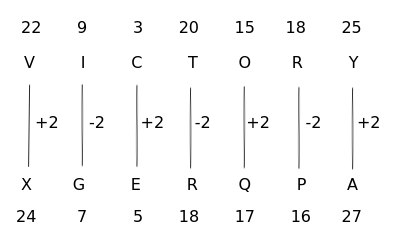Similarly,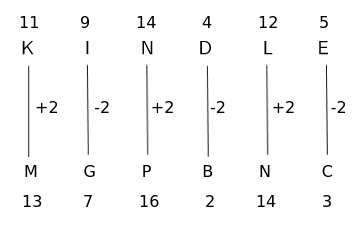$\therefore\$KINDLE is written as MGPBNC in the code language

Hence, the correct answer is Option A

Question 8: In a certain code language, PARK is coded as 6739, ROAD is coded as 3574, POND is coded as 6584 and LANE is coded as 2781. How will LORD be coded as in that language?

a) 2534

b) 7432

c) 5213

d) 5304

Solution:

In the code language,

PARK is coded as 6739

P $\longrightarrow$ 6

A $\longrightarrow$ 7

R $\longrightarrow$ 3

K $\longrightarrow$ 9

R $\longrightarrow$ 3

O $\longrightarrow$ 5

A $\longrightarrow$ 7

D $\longrightarrow$ 4

POND is coded as 6584

P $\longrightarrow$ 6

O $\longrightarrow$ 5

N $\longrightarrow$ 8

D $\longrightarrow$ 4

LANE is coded as 2781

L $\longrightarrow$ 2

A $\longrightarrow$ 7

N $\longrightarrow$ 8

E $\longrightarrow$ 1

Particular values are assigned for different letters.

Similarly, LORD is coded as 2534 in the code language.

Hence, the correct answer is Option A

Question 9: In a certain code language, COBBLER is coded as 130 and TABLE is coded as 93. How will ALMIRAH be coded as in that language?

a) 125

b) 127

c) 129

d) 123

Solution:

Given, COBBLER is coded as 130 and TABLE is coded as 93 in the code language

The logic here is

C opposite is X  $\longrightarrow\$  X = 24

O opposite is L  $\longrightarrow\$  L = 12

B opposite is Y  $\longrightarrow\$  Y = 25

B opposite is Y  $\longrightarrow\$  Y = 25

L opposite is O  $\longrightarrow\$  O = 15

E opposite is V  $\longrightarrow\$  V = 22

R opposite is I $\longrightarrow\$  I = 9

COBBLER  $\longrightarrow\$  XLYYOVI  $\longrightarrow\$  (24 + 12 + 25 + 25 + 15 + 22 + 9) – 2 = 132 – 2 = 130

T opposite is G  $\longrightarrow\$  G = 7

A opposite is Z  $\longrightarrow\$  Z = 26

B opposite is Y  $\longrightarrow\$  Y = 25

L opposite is O  $\longrightarrow\$  O = 15

E opposite is V  $\longrightarrow\$  V = 22

TABLE  $\longrightarrow\$  GZYOV  $\longrightarrow\$  (7 + 26 + 25 + 15 + 22) – 2 = 95 – 2 = 93

The code value is the difference of sum of the values of opposite letters and 2.

Similarly,

A opposite is Z  $\longrightarrow\$  Z = 26

L opposite is O  $\longrightarrow\$  O = 15

M opposite is N  $\longrightarrow\$  N = 14

I opposite is R  $\longrightarrow\$  R = 18

R opposite is I  $\longrightarrow\$  I = 9

A opposite is Z  $\longrightarrow\$  Z = 26

H opposite is S  $\longrightarrow\$  S = 19

The code value for ALMIRAH is (26 + 15 + 14 + 18 + 9 + 26 + 19) – 2 = 127 – 2 = 125

$\therefore\$ALMIRAH is coded as 125 in the code language

Hence, the correct answer is Option A

Question 10: If in a coded language ‘ORAL’ is written as ‘1518112’, then in the same coded language, ‘WRITTEN’ will be written as:

a) 231892020514

b) 221891919514

c) 231892020614

d) 221792020514

Solution:

Given, ORAL is written as 1518112 in the code language

The logic here is

O = 15

R = 18

A = 1

L = 12

The corresponding value of letters is coded in the code language.

Similarly,

W = 23

R = 18

I = 9

T = 20

T = 20

E = 5

N = 14

$\therefore\$ WRITTEN is written as 231892020514 in the code language

Hence, the correct answer is Option A

Question 11: In certain code language, GOGGLE is written as IMIENC. How will PENCIL be written as in that language?

a) RCPAKJ

b) RGPEKN

c) NCLAGJ

d) NGLEGN

Solution:

Given, GOGGLE is written as IMIENC in the code language

The logic here is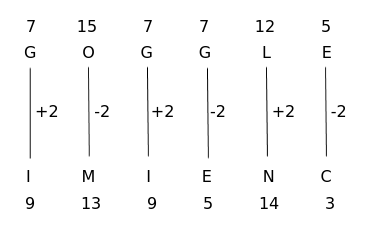Similarly,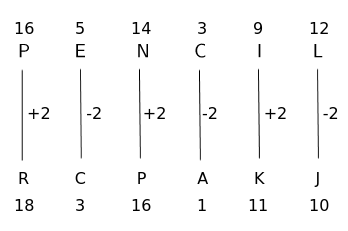$\therefore$ PENCIL is written as RCPAKJ in the code language

Hence, the correct answer is Option A

Question 12: In a certain code language, ‘WARDEN’ is written as ‘SJIWFB’. How will ‘OFFICE’ be written as in that language?

a) JGNKKS

b) KHNJJT

c) JHOKKU

d) JHNKKT

Solution:

Given,  WARDEN is written as SJIWFB in the code language

The logic here is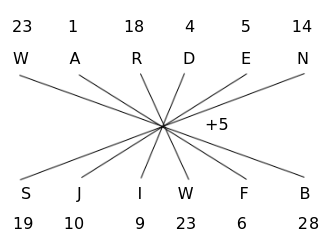Similarly,$\therefore\$OFFICE is written as JHNKKT in the code language

Hence, the correct answer is Option D

Question 13: In a certain code language, ‘SHARP’ is written as ’73’. How will ‘AUDIT’ be written as in the same language?

a) 80

b) 72

c) 76

d) 84

Solution:

Given, SHARP is written as 73 in the code language

The logic here is

S opposite is H  $\longrightarrow\$  H = 8

H opposite is S  $\longrightarrow\$  S = 19

A opposite is Z  $\longrightarrow\$  Z = 26

R opposite is I  $\longrightarrow\$  I = 9

P opposite is K  $\longrightarrow\$  K = 11

SHARP  $\longrightarrow\$  HSZIK $\longrightarrow\$  8 + 19 + 26 + 9 + 11 = 73

The code is the sum of the values of the opposite letters.

Similarly,

A opposite is Z  $\longrightarrow\$  Z = 26

U opposite is F  $\longrightarrow\$  F = 6

D opposite is W  $\longrightarrow\$  W = 23

I opposite is R  $\longrightarrow\$  R = 18

T opposite is G  $\longrightarrow\$  G = 7

The code for AUDIT is 26 + 6 + 23 + 18 + 7 = 80

$\therefore\$AUDIT is written as 80 in the code language

Hence, the correct answer is Option A

Question 14: In a certain code language, MENTION is written as NEITNOM. How will PATTERN be written as in that language?

a) LSOIANN

b) RESWNDU

c) DOEECTS

d) NAETTRP

Solution:

Given, MENTION is written as NEITNOM in the code language

The logic here is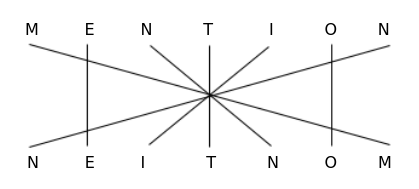Letters are interchanged and remained same alternatively in the code language as shown above

Similarly,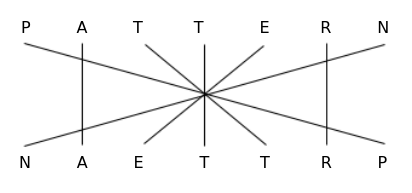$\therefore\$PATTERN is written as NAETTRP in the code language

Hence, the correct answer is Option D

Question 15: In certain code language, BOOK is coded as 139 and PEN is coded as 302. How will COPY be coded as in that language?

a) 2547

b) 167

c) 2822

d) 279

Solution:

Given, BOOK is coded as 139 and PEN is coded as 302 in the code language

K + B = 13 and K – B = 9

P + N = 30 and P – N = 2

The code for word is the combination of sum and difference of values of first and last letters of the word.

Similarly, for COPY

Y + C = 28 and Y – C = 22

$\therefore\$COPY is coded as 2822 in the code language

Hence, the correct answer is Option C

Question 16: In a certain code language, DEAF is written as ‘HJGM’. How will CALM be written as in that language?

a) E B X Y

b) F B X Y

c) G F R T

d) G C Y Z

Solution:

Given, DEAF is written as HJGM in the code language

The logic here is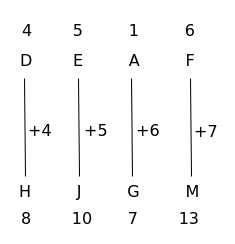Similarly,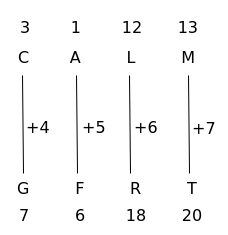$\therefore\$CALM is written as GFRT in code language

Hence, the correct answer is Option C

Question 17: In a certain code language, ‘MARINE’ is written as ‘IRMVEQ’. How will ‘BEAUTY’ be written as in that language?

a) CIGZYD

b) CDOPLY

c) CXYEIF

d) CJHZE

Solution:

Given, MARINE is written as IRMVEQ in the code language

The logic here is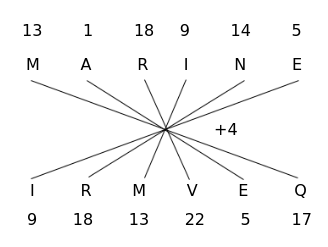Similarly,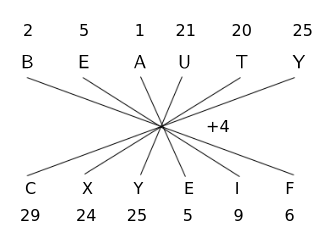$\therefore\$BEAUTY is written as CXYEIF in the code language

Hence, the correct answer is Option C

Question 18: In a certain code language, ‘SURGEON’ is written as ‘5-3-6-17-19-9-10’ then how will ‘DENTIST’ be written in the same code language?

a) 19-18-10-4-15-5-4

b) 23-22-13-7-18-8-7

c) 20-19-10-4-15-6-5

d) 20-19-10-4-15-5-4

Solution:

Given, ‘SURGEON’ is written as ‘5-3-6-17-19-9-10’

The logic here is

S = 19 $\longrightarrow\$ 24 – 19 = 5

U = 21 $\longrightarrow\$ 24 – 21 = 3

R = 18 $\longrightarrow\$ 24 – 18 = 6

G = 7 $\longrightarrow\$ 24 – 7 = 17

E = 5 $\longrightarrow\$ 24 – 5 = 19

O = 15 $\longrightarrow\$ 24 – 15 = 9

N = 14 $\longrightarrow\$ 24 – 14 = 10

The assigned value in the code language is the difference of 24 and respective value of letter.

Similarly,

D = 4 $\longrightarrow\$ 24 – 4 = 20

T= 20 $\longrightarrow\$ 24 – 20 = 4

I = 9 $\longrightarrow\$ 24 – 9 = 15

$\therefore\$DENTIST is written as 20-19-10-4-15-5-4 in the code language.

Hence, the correct answer is Option D

Question 19: In a certain code language, STAR is coded as 55 and CUT is coded as 42. How will ‘PEN’ be coded as in that language?

a) 51

b) 68

c) 55

d) 63

Solution:

Given, STAR is coded as 55 and CUT is coded as 42 in the code language.

The logic here is

S opposite is H $\longrightarrow\$ H = 8

T opposite is G $\longrightarrow\$ G = 7

A opposite is Z $\longrightarrow\$ Z = 26

R opposite is I $\longrightarrow\$ I = 9

STAR $\longrightarrow\$ (8 + 7 + 26 + 9) + 5 = 50 + 5 = 55

C opposite is X $\longrightarrow\$ X = 24

U opposite is F $\longrightarrow\$ F = 6

T opposite is G $\longrightarrow\$ G = 7

CUT $\longrightarrow\$ (24 + 6 + 7) + 5 = 37 + 5 = 42

The code value is the sum of values of opposite letters and 5.

Similarly,

P opposite is K $\longrightarrow\$ K = 11

E opposite is V $\longrightarrow\$ V = 22

N opposite is M $\longrightarrow\$ M = 13

PEN $\longrightarrow\$ (11 + 22 + 13) + 5 = 46 + 5 = 51

$\therefore\$PEN is coded as 51 in the code language.

Hence, the correct answer is Option A

Question 20: In a certain code language, ‘APRICOT’ is written as ‘GLXRIKZ’ then how will ‘ORANGE’ be written in the same code language ?

a) LIZMTV

b) VTNZHM

c) VTMZIL

d) LHZMSV

Solution:

Given, APRICOT is written as GLXRIKZ in the code language

The logic here is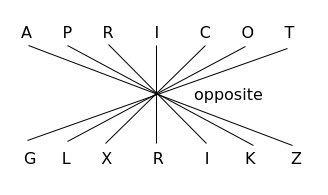The opposite letters are coded as shown in the above pattern.

Similarly,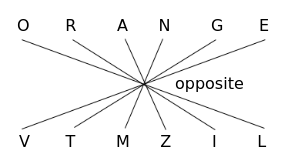$\therefore\$ORANGE is written as VTMZIL in the code language.

Hence, the correct answer is Option C